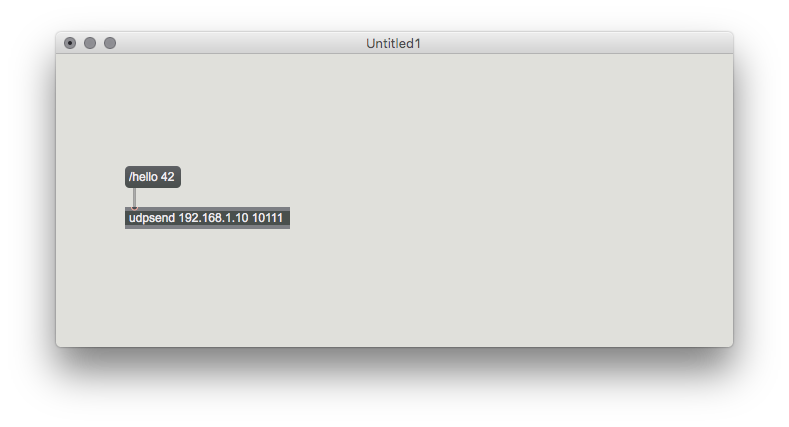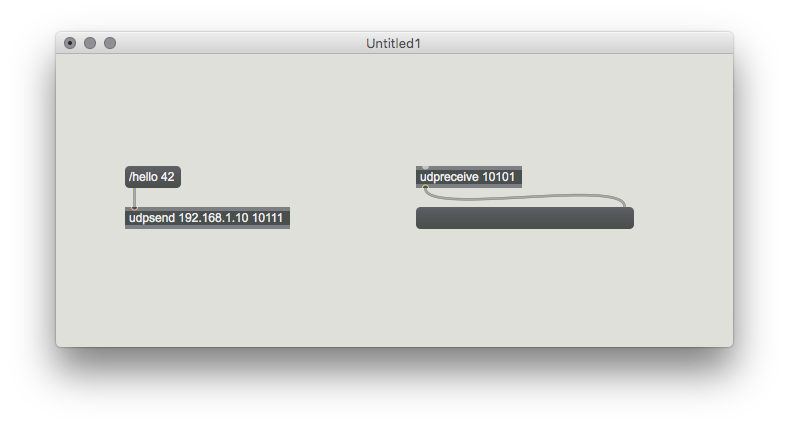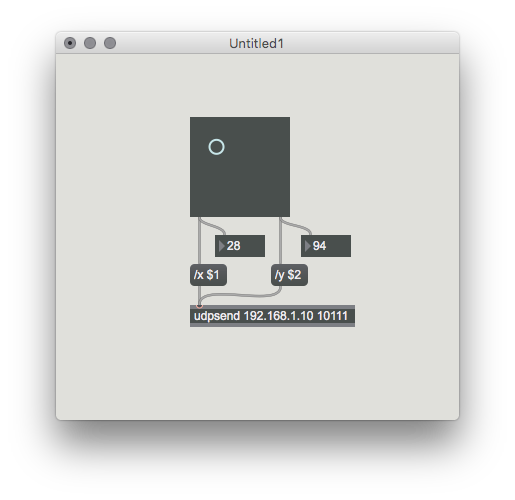# streams

norns studies part 5: system polls, osc, file storage

## numerical superstorm

so far we’ve explored many ways of pushing data around towards musical ends: metronomes, spreadsheet-like tables, midi and grids, even typing commands directly. there is more.

polls report data from the audio subsystem, such as amplitude envelope and pitch detection. to see the available polls:

``````poll.list_names()
``````

and you’ll see:

``````--- polls ---
amp_in_l
amp_in_r
amp_out_l
amp_out_r
cpu_avg
cpu_peak
pitch_in_l
pitch_in_r
------
``````

these are the basic system-wide polls, and engines may add their own polls. for example, softcut adds polls for buffer playback position. let’s set a poll to track the amplitude of input 1:

``````p = poll.set("amp_in_l")
p.callback = function(val) print("in > "..string.format("%.2f",val)) end
p.time = 0.25
p:start()
``````

play some sound into input 1, you’ll see the printed numbers change with the sound level. try changing `p.time` to update the poll interval.

bonus when we want numbers displayed a specific way we can use `string.format`. here we use the format string `"%.2f"` which means we always want two decimals shown. see the printf reference for more formatting methods.

to stop the poll:

``````p:stop()
``````

we can also request a single immediate value from the poll:

``````p:update()
``````

of course instead of just printing out the value of the poll, we should be using it for something more interesting and musical. we’ll do that in the example in the end. but first let’s smash together even more data.

## numbers through air

open sound control (OSC) is a network protocol for sending messages supported by numerous sound and media applications. (OSC also is how lua communicates with supercollider within the norns ecosystem).

OSC messages look like this:

``````/cutoff 500
``````

the first part, `/cutoff` is the path. a series of values and/or strings can come after the path which is the data. in this way an OSC message can be somewhat self-describing: we could assume the message above is to set the cutoff to 500.

we can use OSC in our scripts to interface with the outside world via wifi. for the following example you’ll need to be connected to hotspot or a network. first let’s receieve a message from max/msp:norns scripts listen on OSC port 10111.

this simple max patch sends the message `/hello 42`. you’ll need to change the `udpsend` box to match your norns’ IP address (which you can find in the SYSTEM menu).

sending this messages will print:

``````incoming osc message from	table: 0x169238	/hello
1	42
``````

this is the default callback for OSC. let’s redefine the callback with out own function:

``````function osc_in(path, args, from)
if path == "/hello" then
print("hi!")
elseif path == "/x" then
x = args
elseif path == "/y" then
y = args
elseif path == "/xy" then
x = args
y = args
else
print(path)
tab.print(args)
end
print("osc from " .. from .. " port " .. from)
end

osc.event = osc_in
``````

try sending osc messages with `/x` and `/y` as paths and a single number as data. path `/xy` will accept two numbers and set both values. this is how we map OSC paths to functionality within our script.

we can also extract the address and port of the sender. note that the receiving port will typically be different, so check the ports of each OSC client. let’s send a message using our script back to max:above we set up a receive port on 10101. here’s how we send to it:

``````dest = {"192.168.1.12",10101}
osc.send(dest, "/soup", {1,10})
``````

`dest` is the destination we’re sending to, so change the IP address to match the address where you received the messages earlier. the second argument is the path, followed by a table (curly brakcets) with the data. even if you want to send a single value, it still has to be inside a table.

norns is also auto-discoverable as an OSC device. for example, using touchOSC is very straightforward as “norns” should show up in the config list if both are connected to the same network.

## long term number storage

there will come a time when you have collected too many numbers, and they are precious and you want to save them for later. norns has a filesystem that can store a ton of numbers. here’s the easy way:

``````my_secret_bits = {2,-1,21,0}
tab.save(my_secret_bits, _path.data.."secret.txt")
``````

`tab.save` is a function which saves a table to disk. we specify the file as `secret.txt` inside the folder `_path.data` (which is a global for `/home/we/dust/data/`).

let’s now load the same file to a different table:

``````summoned_bits = tab.load(_path.data.."secret.txt")
``````

a quick check via `tab.print(summoned_bits)` will show that the read was successful:

``````1	2
2	-1
3	21
4	0
``````

let’s do some more complex file operations. here’s how you get a folder listing:

``````listing = util.scandir(paths.home)
tab.print(listing)
``````

you’ll see something resembling this:

``````1    bin/
2    dust/
3    maiden/
4    norns/
5    norns-image/
6    update/
7    changelog.txt
8    version.txt
``````

`util.scandir` takes one argument which is a folder path, and then it returns a table the folder contents. let’s load one of these files and print it out, just to see how file loading works:

``````function print_file(filepath)
local f=io.open(filepath,"r")
if f==nil then
else
f:close()
for line in io.lines(filepath) do
-- this is where you would do something useful!
-- but for now we'll just print each line
print(line)
end
end
end

-- let's test it:
folder = paths.home.."/"
listing = util.scandir(folder)
print_file(folder..listing)

``````

the file `changelog.txt` should be printed! stepping through `print_file`:

• argument is a file with path
• checks if the file exists
• uses a `for` loop to iterate on each line of the file

writing a file is not much more complex:

``````f=io.open(_path.data .. "other_test.txt","w+")
f:write("dear diary,\n")
f:write("10011010\n")
f:close(f)
``````

## example: streams

putting together concepts above. this script is demonstrated in the video up top.

here’s a max patch that uses a `pictslider` object for 2d control. the script is also compatible with the touchOSC “simple” template.``````-- streams
-- norns study 5
--
-- KEY2 - clear pitch table
-- KEY3 - capture pitch to table
--
-- OSC patterns:
-- /x i : noise 0-127
-- /y i : cut 0-127
-- /3/xy f f : noise 0-1 cut 0-1

engine.name = 'PolySub'

collection = {}
last = -1

function init()
screen.level(4)
screen.aa(0)
screen.line_width(1)

params:set_action("shape", function(x) engine.shape(x) end)
params:set_action("timbre", function(x) engine.timbre(x) end)
params:set_action("noise", function(x) engine.noise(x) end)
params:set_action("cut", function(x) engine.cut(x) end)
params:set_action("fgain", function(x) engine.fgain(x) end)
params:set_action("cutEnvAmt", function(x) engine.cutEnvAmt(x) end)
params:set_action("detune", function(x) engine.detune(x) end)
params:set_action("ampAtk", function(x) engine.ampAtk(x) end)
params:set_action("ampDec", function(x) engine.ampDec(x) end)
params:set_action("ampSus", function(x) engine.ampSus(x) end)
params:set_action("ampRel", function(x) engine.ampRel(x) end)
params:set_action("cutAtk", function(x) engine.cutAtk(x) end)
params:set_action("cutDec", function(x) engine.cutDec(x) end)
params:set_action("cutSus", function(x) engine.cutSus(x) end)
params:set_action("cutRel", function(x) engine.cutRel(x) end)
params:bang()

engine.level(0.02)

pitch_tracker = poll.set("pitch_in_l")
pitch_tracker.callback = function(x)
if x > 0 then
table.insert(collection,x)
engine.start(#collection,x)
last = x
redraw()
end
end
end

function key(n,z)
if n==2 and z==1 then
engine.stopAll()
collection = {}
last = -1
redraw()
elseif n==3 and z==1 and #collection < 16 then
pitch_tracker:update()
end
end

local osc_in = function(path, args, from)
if path == "/x" then
params:set_raw("noise",args/127)
elseif path == "/y" then
params:set_raw("cut",args/127)
elseif path == "/3/xy" then
params:set_raw("noise",args)
params:set_raw("cut",1-args)
else
print(path)
tab.print(args)
end
end

osc.event = osc_in

function redraw()
screen.clear()
screen.move(0,10)
if last ~= -1 then screen.text(#collection .. " > " .. string.format("%.2f",last)) end
for i,y in pairs(collection) do
screen.move(4+(i-1)*8,60)
screen.line_rel(0,-(8 * (math.log(collection[i]))-30))
screen.stroke()
end
screen.update()
end
``````

## continued

• part 1: many tomorrows // variables, simple maths, keys + encoders
• part 2: patterning // screen drawing, for/while loops, tables
• part 3: spacetime // functions, parameters, time
• part 4: physical // grids + midi
• part 5: streams
• further: softcut studies // a multi-voice sample playback and recording system built into norns

## community

ask questions and share what you’re making at llllllll.co

edits to this study welcome, see monome/docs